Скачать презентацию Fall 2011 Part 8 Input Modeling CSC 446 546

0cae01a88faf8d3c5f7ee9e1b48a885e.ppt

• Количество слайдов: 44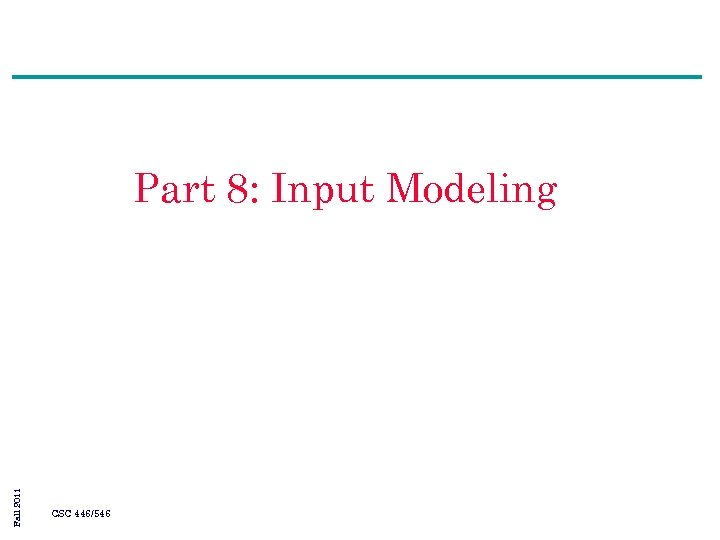Fall 2011 Part 8: Input Modeling CSC 446/546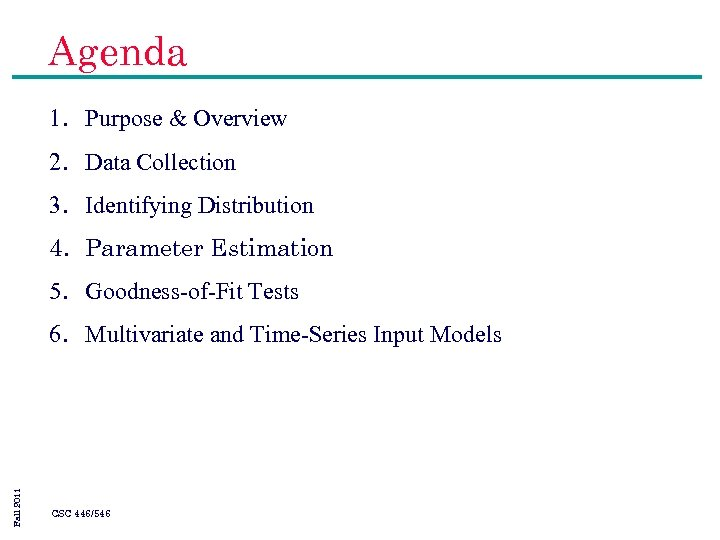Agenda 1. Purpose & Overview 2. Data Collection 3. Identifying Distribution 4. Parameter Estimation 5. Goodness-of-Fit Tests Fall 2011 6. Multivariate and Time-Series Input Models CSC 446/546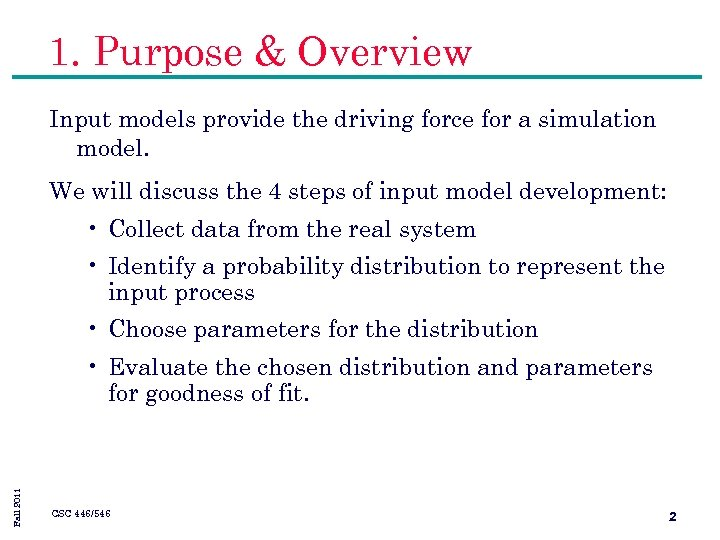1. Purpose & Overview Input models provide the driving force for a simulation model. We will discuss the 4 steps of input model development: • Collect data from the real system • Identify a probability distribution to represent the input process • Choose parameters for the distribution Fall 2011 • Evaluate the chosen distribution and parameters for goodness of fit. CSC 446/546 2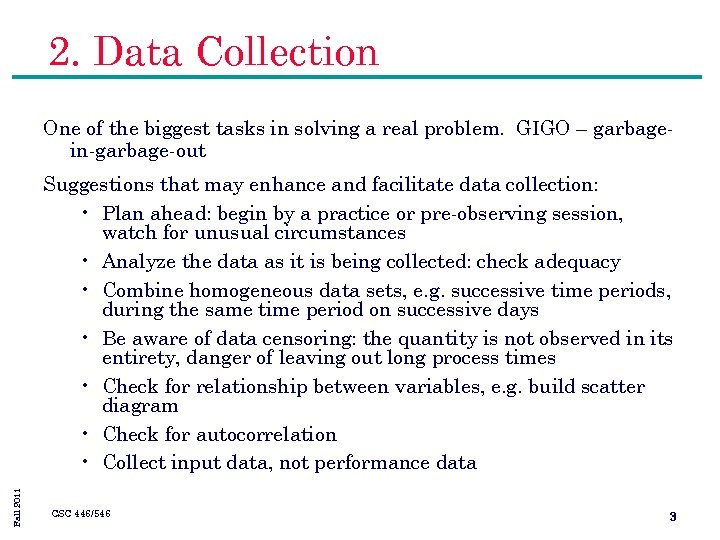2. Data Collection One of the biggest tasks in solving a real problem. GIGO – garbagein-garbage-out Fall 2011 Suggestions that may enhance and facilitate data collection: • Plan ahead: begin by a practice or pre-observing session, watch for unusual circumstances • Analyze the data as it is being collected: check adequacy • Combine homogeneous data sets, e. g. successive time periods, during the same time period on successive days • Be aware of data censoring: the quantity is not observed in its entirety, danger of leaving out long process times • Check for relationship between variables, e. g. build scatter diagram • Check for autocorrelation • Collect input data, not performance data CSC 446/546 3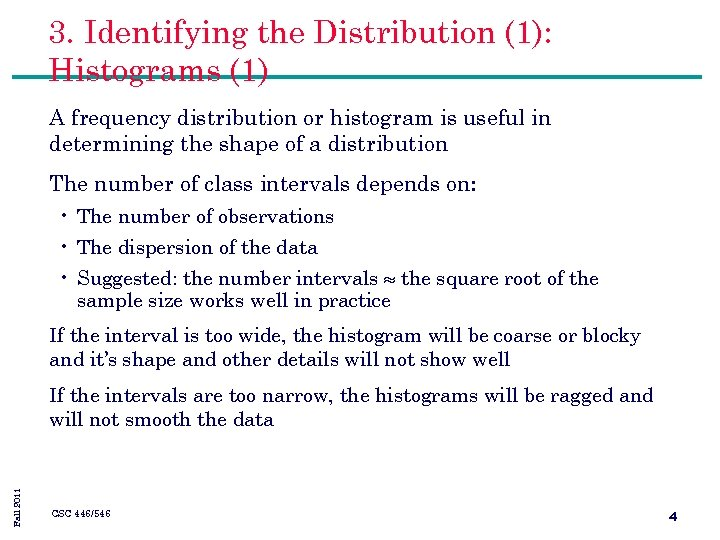3. Identifying the Distribution (1): Histograms (1) A frequency distribution or histogram is useful in determining the shape of a distribution The number of class intervals depends on: • The number of observations • The dispersion of the data • Suggested: the number intervals the square root of the sample size works well in practice If the interval is too wide, the histogram will be coarse or blocky and it’s shape and other details will not show well Fall 2011 If the intervals are too narrow, the histograms will be ragged and will not smooth the data CSC 446/546 4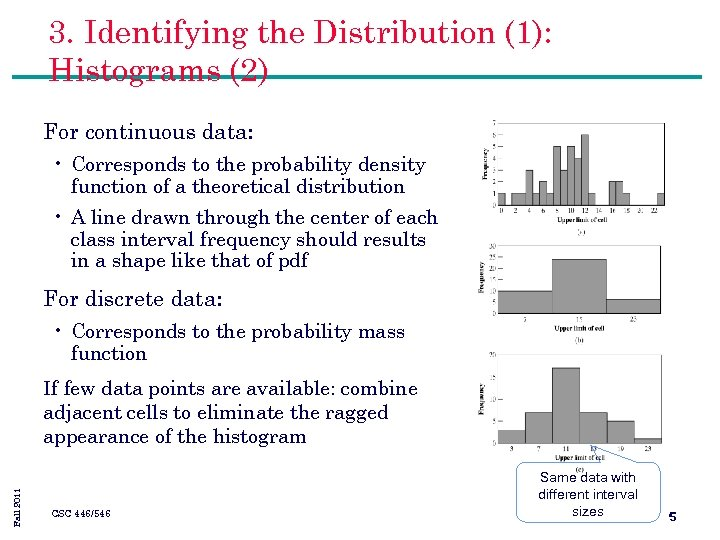3. Identifying the Distribution (1): Histograms (2) For continuous data: • Corresponds to the probability density function of a theoretical distribution • A line drawn through the center of each class interval frequency should results in a shape like that of pdf For discrete data: • Corresponds to the probability mass function Fall 2011 If few data points are available: combine adjacent cells to eliminate the ragged appearance of the histogram CSC 446/546 Same data with different interval sizes 5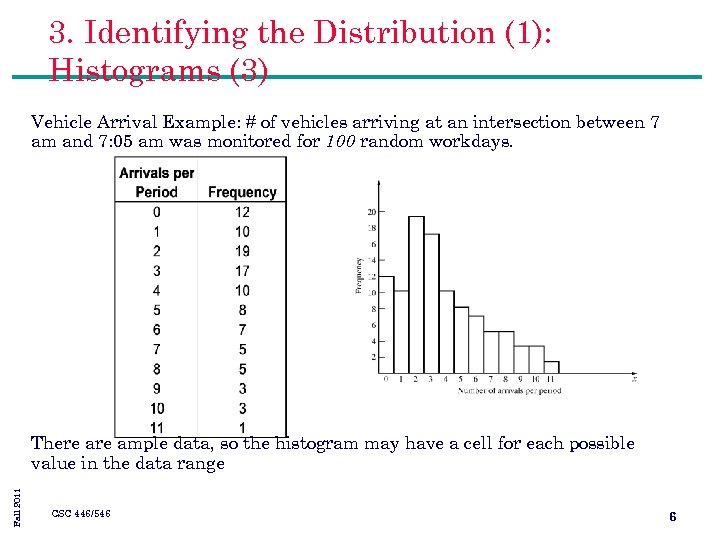3. Identifying the Distribution (1): Histograms (3) Vehicle Arrival Example: # of vehicles arriving at an intersection between 7 am and 7: 05 am was monitored for 100 random workdays. Fall 2011 There ample data, so the histogram may have a cell for each possible value in the data range CSC 446/546 6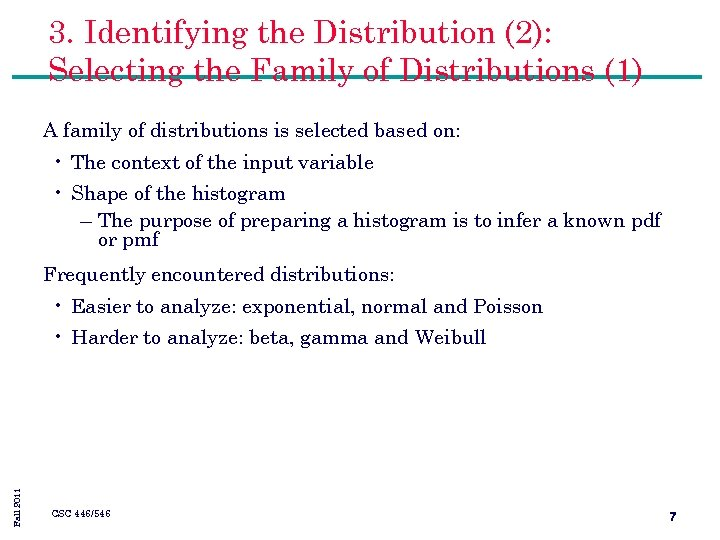3. Identifying the Distribution (2): Selecting the Family of Distributions (1) A family of distributions is selected based on: • The context of the input variable • Shape of the histogram – The purpose of preparing a histogram is to infer a known pdf or pmf Frequently encountered distributions: • Easier to analyze: exponential, normal and Poisson Fall 2011 • Harder to analyze: beta, gamma and Weibull CSC 446/546 7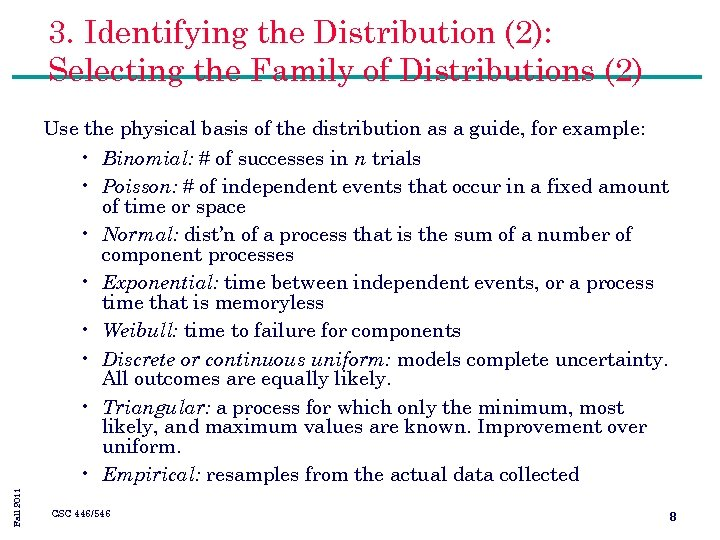3. Identifying the Distribution (2): Selecting the Family of Distributions (2) Fall 2011 Use the physical basis of the distribution as a guide, for example: • Binomial: # of successes in n trials • Poisson: # of independent events that occur in a fixed amount of time or space • Normal: dist’n of a process that is the sum of a number of component processes • Exponential: time between independent events, or a process time that is memoryless • Weibull: time to failure for components • Discrete or continuous uniform: models complete uncertainty. All outcomes are equally likely. • Triangular: a process for which only the minimum, most likely, and maximum values are known. Improvement over uniform. • Empirical: resamples from the actual data collected CSC 446/546 8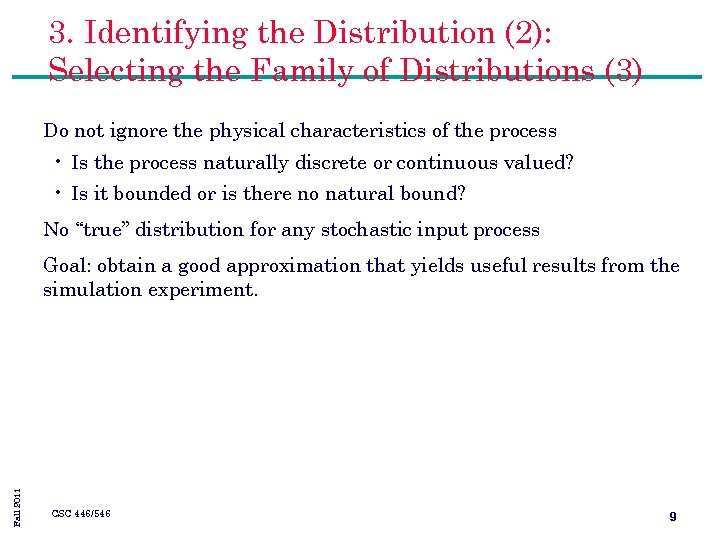3. Identifying the Distribution (2): Selecting the Family of Distributions (3) Do not ignore the physical characteristics of the process • Is the process naturally discrete or continuous valued? • Is it bounded or is there no natural bound? No “true” distribution for any stochastic input process Fall 2011 Goal: obtain a good approximation that yields useful results from the simulation experiment. CSC 446/546 9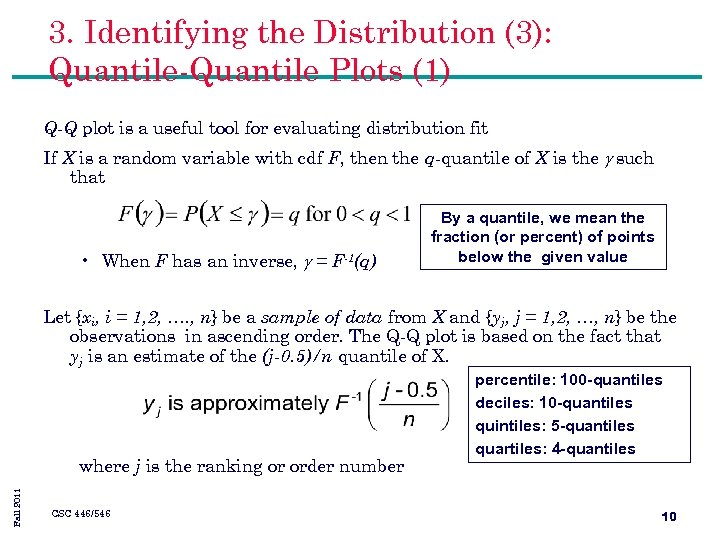3. Identifying the Distribution (3): Quantile-Quantile Plots (1) Q-Q plot is a useful tool for evaluating distribution fit If X is a random variable with cdf F, then the q-quantile of X is the g such that • When F has an inverse, g = F-1(q) By a quantile, we mean the fraction (or percent) of points below the given value Let {xi, i = 1, 2, …. , n} be a sample of data from X and {yj, j = 1, 2, …, n} be the observations in ascending order. The Q-Q plot is based on the fact that yj is an estimate of the (j-0. 5)/n quantile of X. Fall 2011 where j is the ranking or order number CSC 446/546 percentile: 100 -quantiles deciles: 10 -quantiles quintiles: 5 -quantiles quartiles: 4 -quantiles 10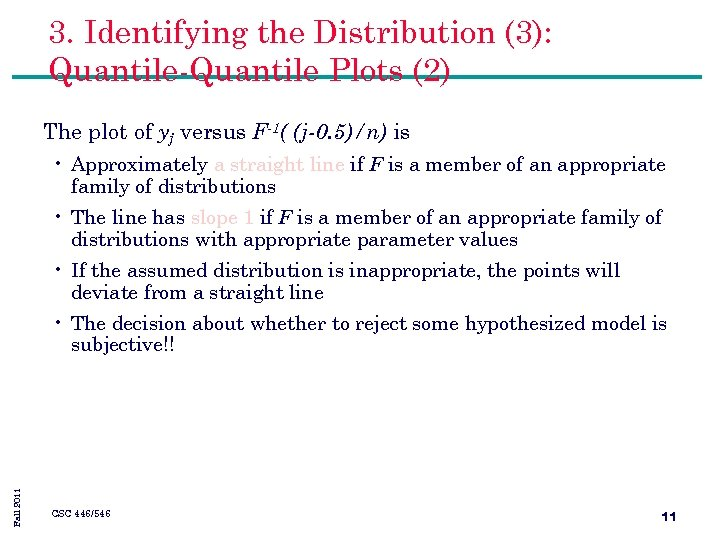3. Identifying the Distribution (3): Quantile-Quantile Plots (2) The plot of yj versus F-1( (j-0. 5)/n) is • Approximately a straight line if F is a member of an appropriate family of distributions • The line has slope 1 if F is a member of an appropriate family of distributions with appropriate parameter values • If the assumed distribution is inappropriate, the points will deviate from a straight line Fall 2011 • The decision about whether to reject some hypothesized model is subjective!! CSC 446/546 11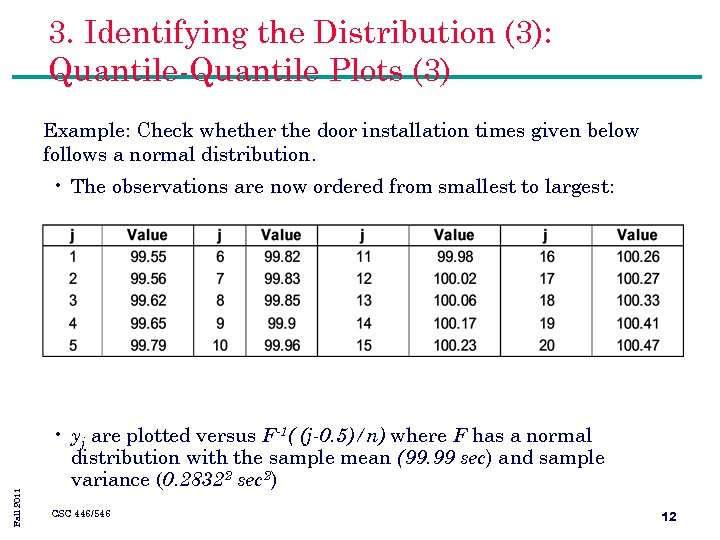3. Identifying the Distribution (3): Quantile-Quantile Plots (3) Example: Check whether the door installation times given below follows a normal distribution. Fall 2011 • The observations are now ordered from smallest to largest: • yj are plotted versus F-1( (j-0. 5)/n) where F has a normal distribution with the sample mean (99. 99 sec) and sample variance (0. 28322 sec 2) CSC 446/546 12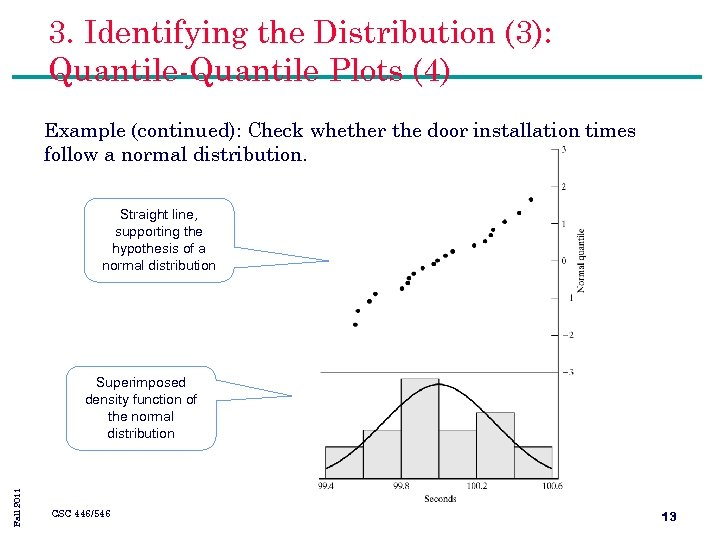3. Identifying the Distribution (3): Quantile-Quantile Plots (4) Example (continued): Check whether the door installation times follow a normal distribution. Straight line, supporting the hypothesis of a normal distribution Fall 2011 Superimposed density function of the normal distribution CSC 446/546 13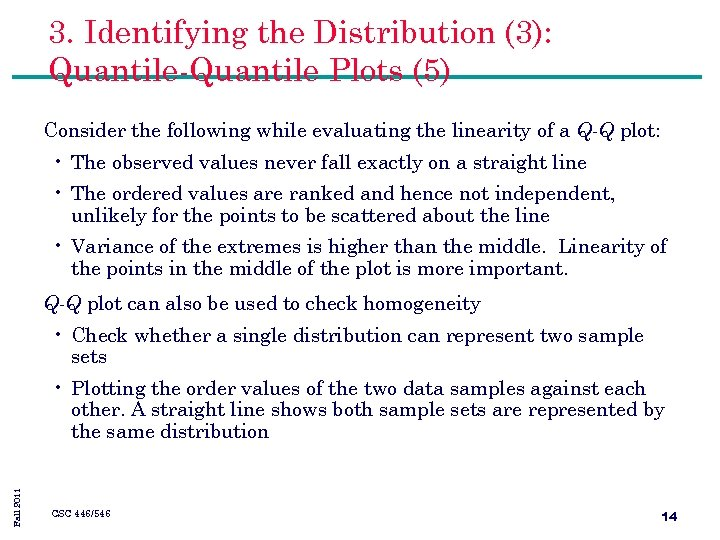3. Identifying the Distribution (3): Quantile-Quantile Plots (5) Consider the following while evaluating the linearity of a Q-Q plot: • The observed values never fall exactly on a straight line • The ordered values are ranked and hence not independent, unlikely for the points to be scattered about the line • Variance of the extremes is higher than the middle. Linearity of the points in the middle of the plot is more important. Q-Q plot can also be used to check homogeneity • Check whether a single distribution can represent two sample sets Fall 2011 • Plotting the order values of the two data samples against each other. A straight line shows both sample sets are represented by the same distribution CSC 446/546 14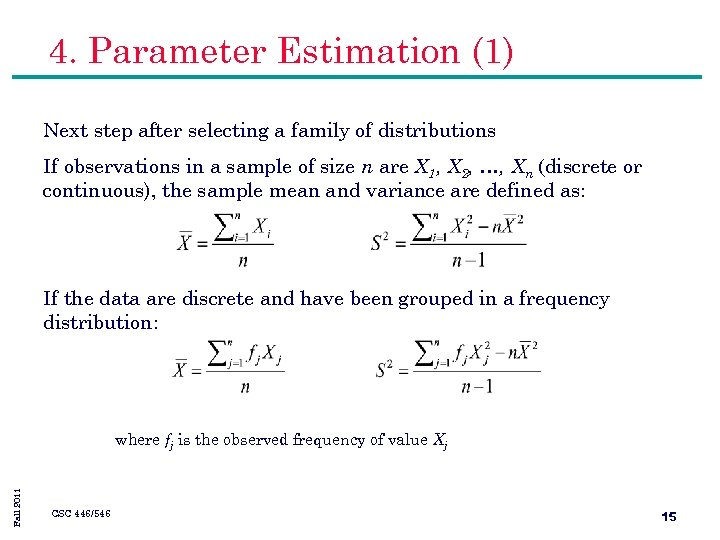4. Parameter Estimation (1) Next step after selecting a family of distributions If observations in a sample of size n are X 1, X 2, …, Xn (discrete or continuous), the sample mean and variance are defined as: If the data are discrete and have been grouped in a frequency distribution: Fall 2011 where fj is the observed frequency of value Xj CSC 446/546 15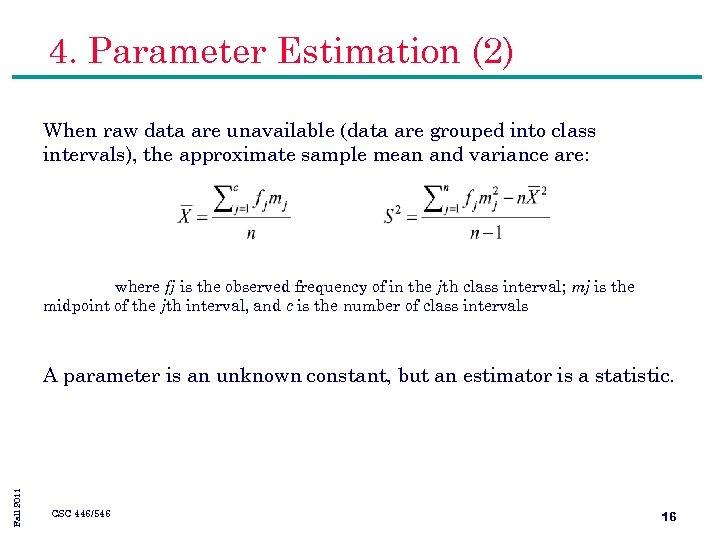4. Parameter Estimation (2) When raw data are unavailable (data are grouped into class intervals), the approximate sample mean and variance are: where fj is the observed frequency of in the jth class interval; mj is the midpoint of the jth interval, and c is the number of class intervals Fall 2011 A parameter is an unknown constant, but an estimator is a statistic. CSC 446/546 16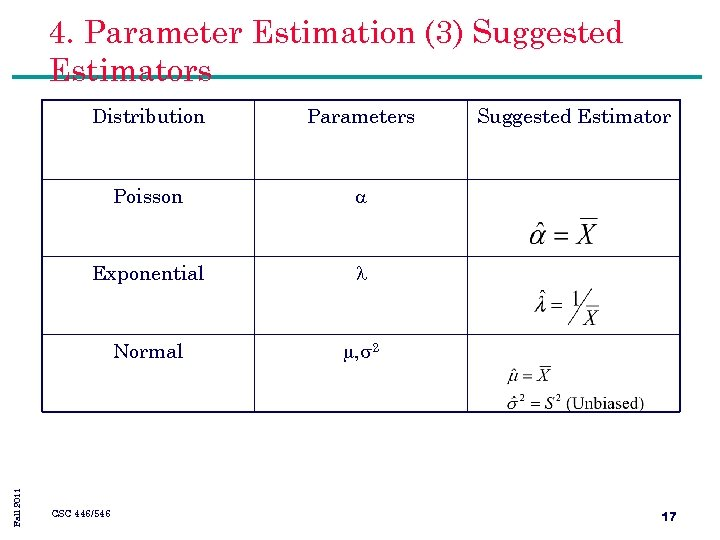4. Parameter Estimation (3) Suggested Estimators Parameters Poisson Exponential Normal Fall 2011 Distribution , 2 CSC 446/546 Suggested Estimator 17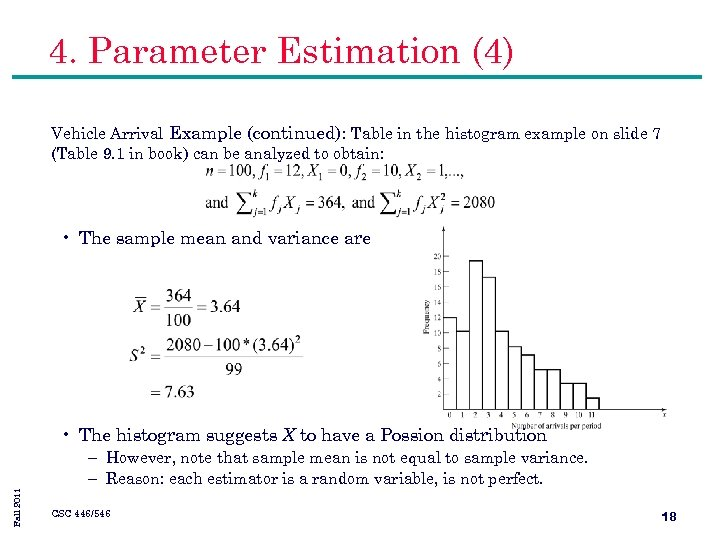4. Parameter Estimation (4) Vehicle Arrival Example (continued): Table in the histogram example on slide 7 (Table 9. 1 in book) can be analyzed to obtain: • The sample mean and variance are • The histogram suggests X to have a Possion distribution Fall 2011 – However, note that sample mean is not equal to sample variance. – Reason: each estimator is a random variable, is not perfect. CSC 446/546 18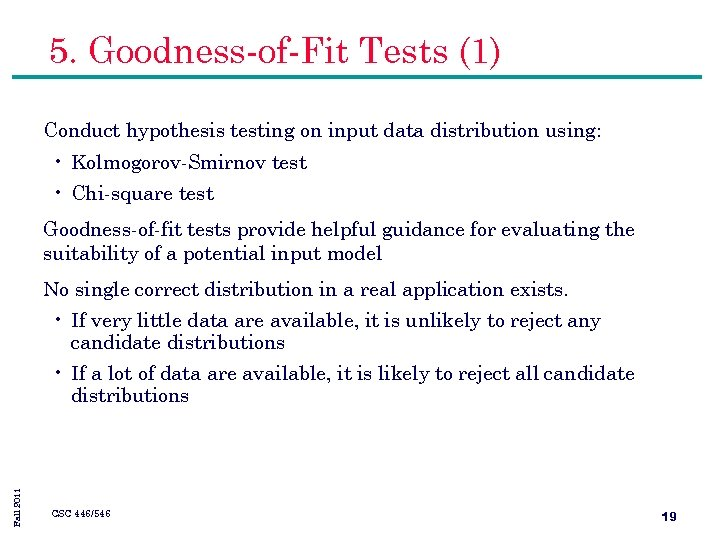5. Goodness-of-Fit Tests (1) Conduct hypothesis testing on input data distribution using: • Kolmogorov-Smirnov test • Chi-square test Goodness-of-fit tests provide helpful guidance for evaluating the suitability of a potential input model No single correct distribution in a real application exists. • If very little data are available, it is unlikely to reject any candidate distributions Fall 2011 • If a lot of data are available, it is likely to reject all candidate distributions CSC 446/546 19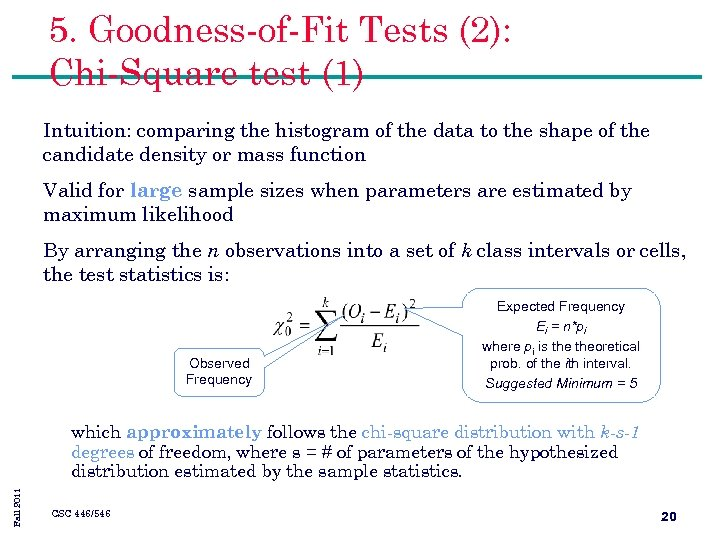5. Goodness-of-Fit Tests (2): Chi-Square test (1) Intuition: comparing the histogram of the data to the shape of the candidate density or mass function Valid for large sample sizes when parameters are estimated by maximum likelihood By arranging the n observations into a set of k class intervals or cells, the test statistics is: Observed Frequency Expected Frequency Ei = n*pi where pi is theoretical prob. of the ith interval. Suggested Minimum = 5 Fall 2011 which approximately follows the chi-square distribution with k-s-1 degrees of freedom, where s = # of parameters of the hypothesized distribution estimated by the sample statistics. CSC 446/546 20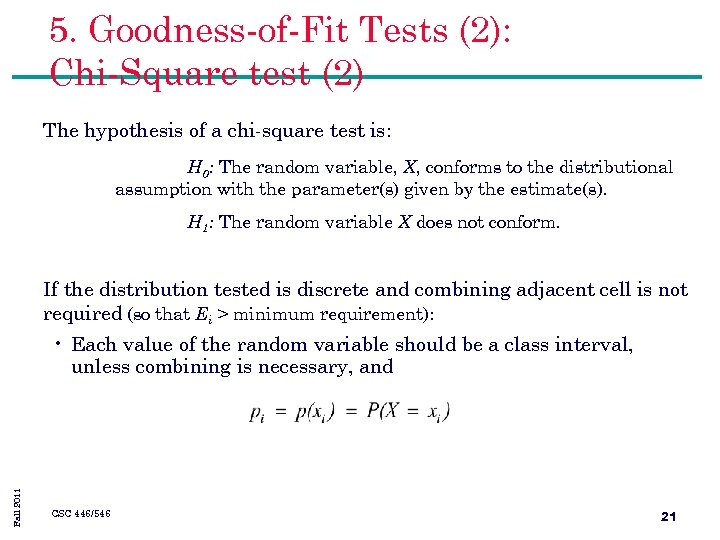5. Goodness-of-Fit Tests (2): Chi-Square test (2) The hypothesis of a chi-square test is: H 0: The random variable, X, conforms to the distributional assumption with the parameter(s) given by the estimate(s). H 1: The random variable X does not conform. If the distribution tested is discrete and combining adjacent cell is not required (so that Ei > minimum requirement): Fall 2011 • Each value of the random variable should be a class interval, unless combining is necessary, and CSC 446/546 21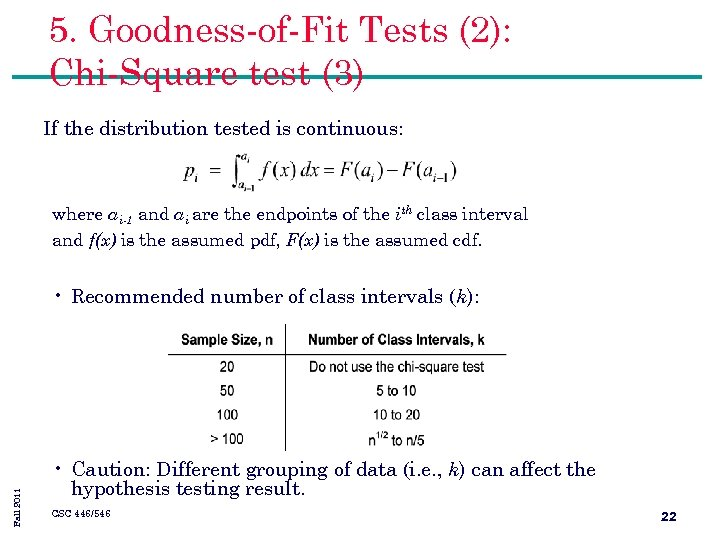5. Goodness-of-Fit Tests (2): Chi-Square test (3) If the distribution tested is continuous: where ai-1 and ai are the endpoints of the ith class interval and f(x) is the assumed pdf, F(x) is the assumed cdf. Fall 2011 • Recommended number of class intervals (k): • Caution: Different grouping of data (i. e. , k) can affect the hypothesis testing result. CSC 446/546 22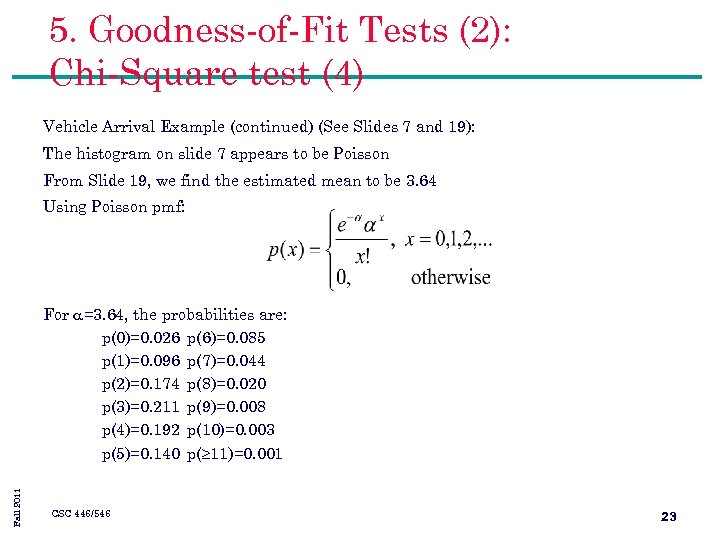5. Goodness-of-Fit Tests (2): Chi-Square test (4) Vehicle Arrival Example (continued) (See Slides 7 and 19): The histogram on slide 7 appears to be Poisson From Slide 19, we find the estimated mean to be 3. 64 Using Poisson pmf: Fall 2011 For =3. 64, the probabilities are: p(0)=0. 026 p(6)=0. 085 p(1)=0. 096 p(7)=0. 044 p(2)=0. 174 p(8)=0. 020 p(3)=0. 211 p(9)=0. 008 p(4)=0. 192 p(10)=0. 003 p(5)=0. 140 p( 11)=0. 001 CSC 446/546 23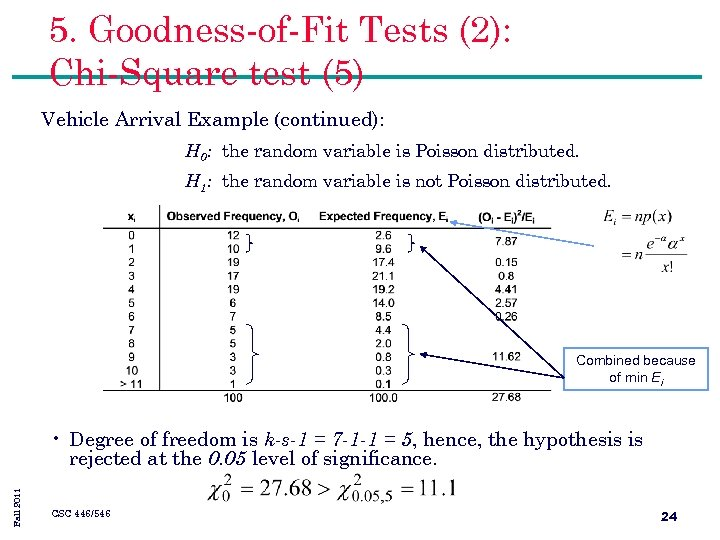5. Goodness-of-Fit Tests (2): Chi-Square test (5) Vehicle Arrival Example (continued): H 0: the random variable is Poisson distributed. H 1: the random variable is not Poisson distributed. Combined because of min Ei Fall 2011 • Degree of freedom is k-s-1 = 7 -1 -1 = 5, hence, the hypothesis is rejected at the 0. 05 level of significance. CSC 446/546 24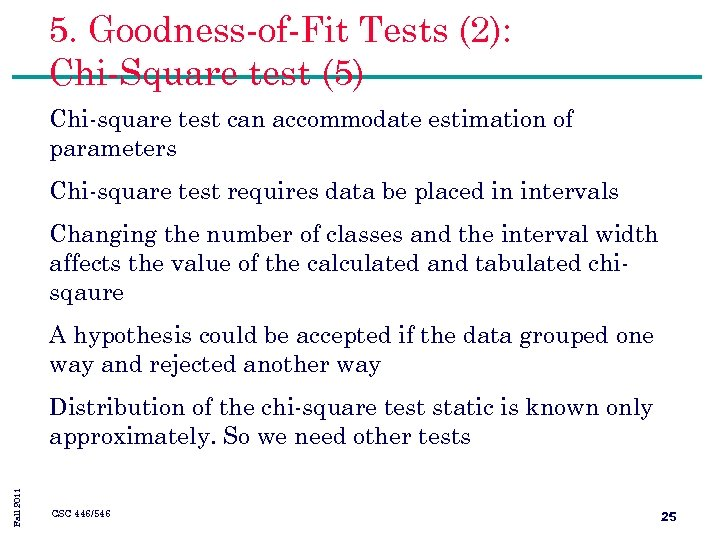5. Goodness-of-Fit Tests (2): Chi-Square test (5) Chi-square test can accommodate estimation of parameters Chi-square test requires data be placed in intervals Changing the number of classes and the interval width affects the value of the calculated and tabulated chisqaure A hypothesis could be accepted if the data grouped one way and rejected another way Fall 2011 Distribution of the chi-square test static is known only approximately. So we need other tests CSC 446/546 25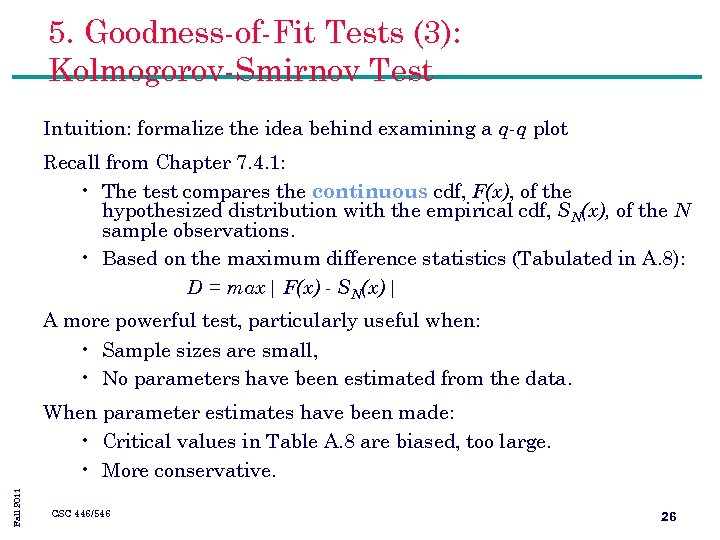5. Goodness-of-Fit Tests (3): Kolmogorov-Smirnov Test Intuition: formalize the idea behind examining a q-q plot Recall from Chapter 7. 4. 1: • The test compares the continuous cdf, F(x), of the hypothesized distribution with the empirical cdf, SN(x), of the N sample observations. • Based on the maximum difference statistics (Tabulated in A. 8): D = max| F(x) - SN(x)| A more powerful test, particularly useful when: • Sample sizes are small, • No parameters have been estimated from the data. Fall 2011 When parameter estimates have been made: • Critical values in Table A. 8 are biased, too large. • More conservative. CSC 446/546 26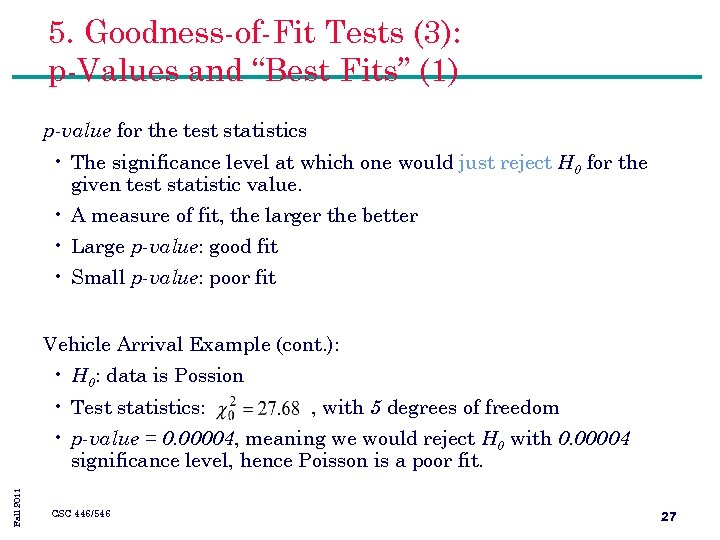5. Goodness-of-Fit Tests (3): p-Values and “Best Fits” (1) p-value for the test statistics • The significance level at which one would just reject H 0 for the given test statistic value. • A measure of fit, the larger the better • Large p-value: good fit • Small p-value: poor fit Vehicle Arrival Example (cont. ): • H 0: data is Possion • Test statistics: , with 5 degrees of freedom Fall 2011 • p-value = 0. 00004, meaning we would reject H 0 with 0. 00004 significance level, hence Poisson is a poor fit. CSC 446/546 275. Goodness-of-Fit Tests (3): p-Values and “Best Fits” (2) Many software use p-value as the ranking measure to automatically determine the “best fit”. • Software could fit every distribution at our disposal, compute the test statistic for each fit and choose the distribution that yields largest p-value. Things to be cautious about: • Software may not know about the physical basis of the data, distribution families it suggests may be inappropriate. • Close conformance to the data does not always lead to the most appropriate input model. • p-value does not say much about where the lack of fit occurs Fall 2011 Recommended: always inspect the automatic selection using graphical methods. CSC 446/546 28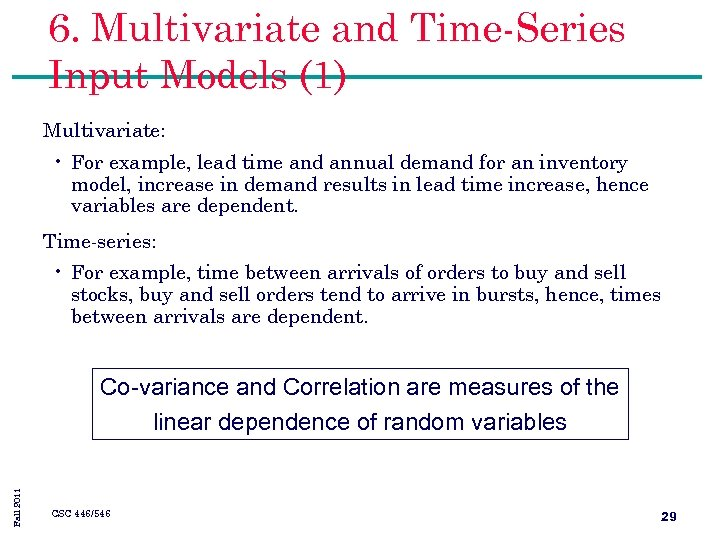6. Multivariate and Time-Series Input Models (1) Multivariate: • For example, lead time and annual demand for an inventory model, increase in demand results in lead time increase, hence variables are dependent. Time-series: • For example, time between arrivals of orders to buy and sell stocks, buy and sell orders tend to arrive in bursts, hence, times between arrivals are dependent. Fall 2011 Co-variance and Correlation are measures of the linear dependence of random variables CSC 446/546 29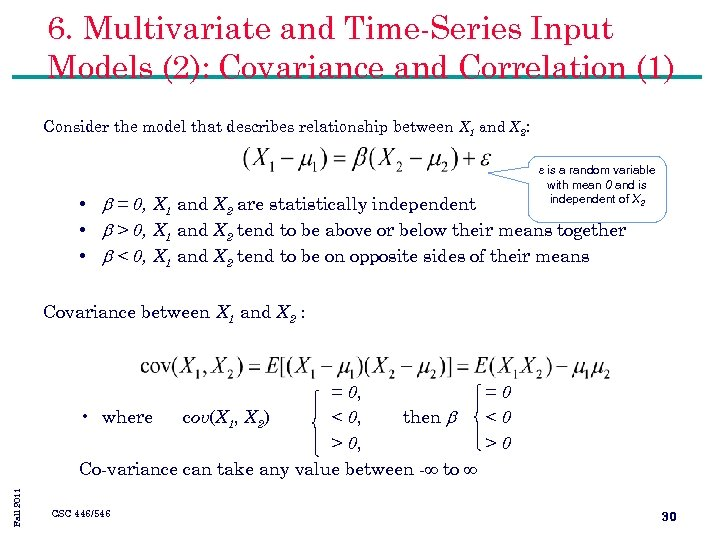6. Multivariate and Time-Series Input Models (2): Covariance and Correlation (1) Consider the model that describes relationship between X 1 and X 2: e is a random variable with mean 0 and is independent of X 2 • b = 0, X 1 and X 2 are statistically independent • b > 0, X 1 and X 2 tend to be above or below their means together • b < 0, X 1 and X 2 tend to be on opposite sides of their means Covariance between X 1 and X 2 : Fall 2011 = 0, =0 • where cov(X 1, X 2) < 0, then b <0 > 0, >0 Co-variance can take any value between - to CSC 446/546 30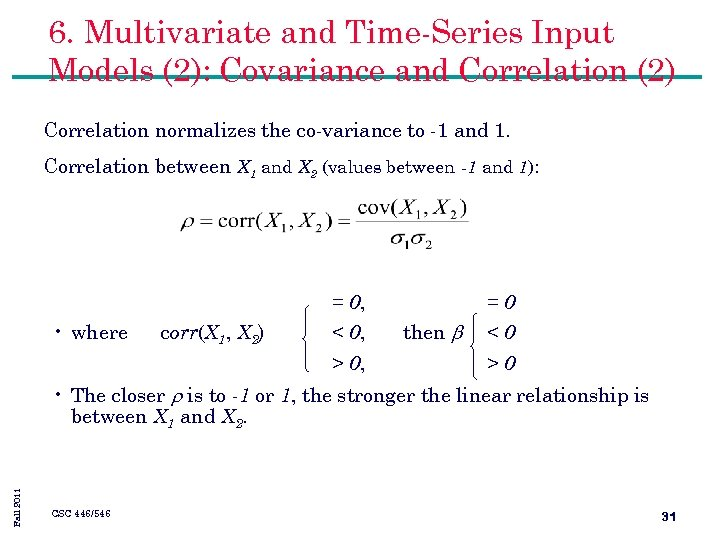6. Multivariate and Time-Series Input Models (2): Covariance and Correlation (2) Correlation normalizes the co-variance to -1 and 1. Correlation between X 1 and X 2 (values between -1 and 1): = 0, • where corr(X 1, X 2) < 0, > 0, =0 then b <0 >0 Fall 2011 • The closer is to -1 or 1, the stronger the linear relationship is between X 1 and X 2. CSC 446/546 31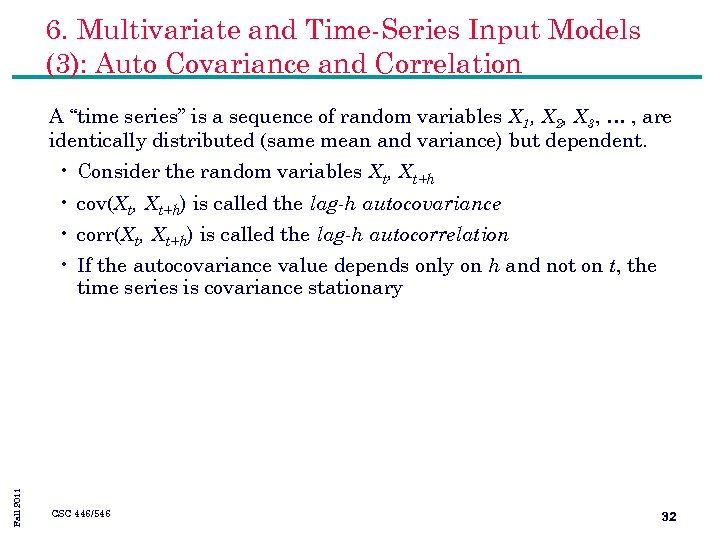6. Multivariate and Time-Series Input Models (3): Auto Covariance and Correlation A “time series” is a sequence of random variables X 1, X 2, X 3, … , are identically distributed (same mean and variance) but dependent. • Consider the random variables Xt, Xt+h • cov(Xt, Xt+h) is called the lag-h autocovariance • corr(Xt, Xt+h) is called the lag-h autocorrelation Fall 2011 • If the autocovariance value depends only on h and not on t, the time series is covariance stationary CSC 446/546 32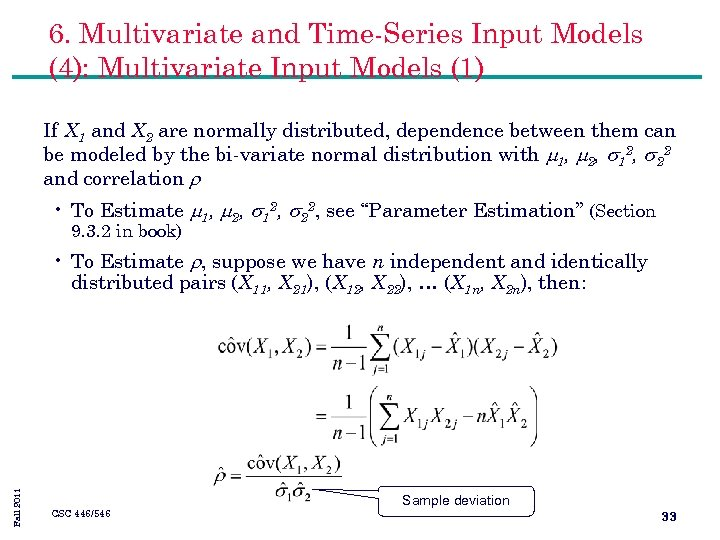6. Multivariate and Time-Series Input Models (4): Multivariate Input Models (1) If X 1 and X 2 are normally distributed, dependence between them can be modeled by the bi-variate normal distribution with 1, 2, s 12, s 22 and correlation • To Estimate 1, 2, s 12, s 22, see “Parameter Estimation” (Section 9. 3. 2 in book) Fall 2011 • To Estimate , suppose we have n independent and identically distributed pairs (X 11, X 21), (X 12, X 22), … (X 1 n, X 2 n), then: CSC 446/546 Sample deviation 33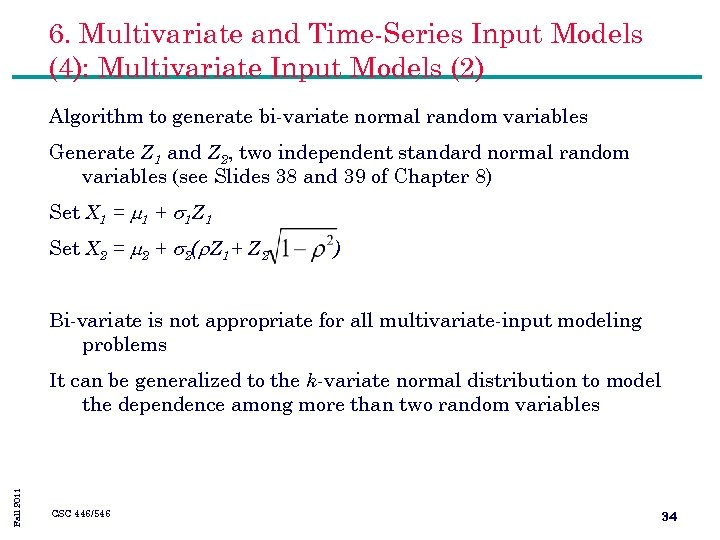6. Multivariate and Time-Series Input Models (4): Multivariate Input Models (2) Algorithm to generate bi-variate normal random variables Generate Z 1 and Z 2, two independent standard normal random variables (see Slides 38 and 39 of Chapter 8) Set X 1 = 1 + s 1 Z 1 Set X 2 = 2 + s 2( Z 1+ Z 2 ) Bi-variate is not appropriate for all multivariate-input modeling problems Fall 2011 It can be generalized to the k-variate normal distribution to model the dependence among more than two random variables CSC 446/546 34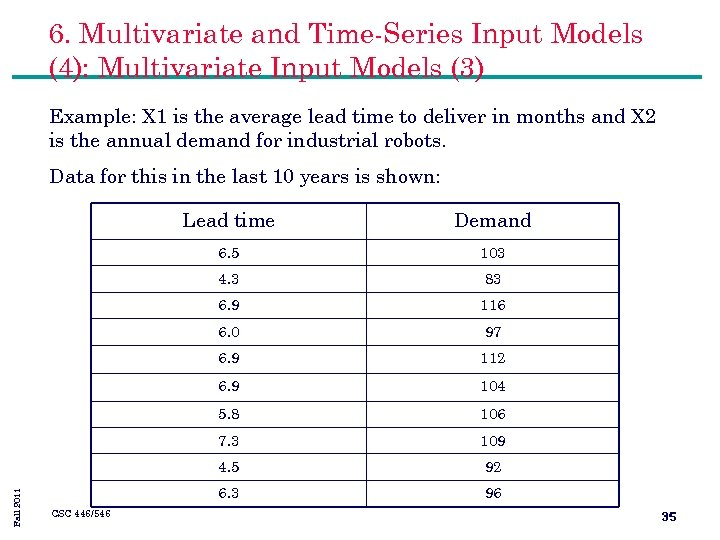6. Multivariate and Time-Series Input Models (4): Multivariate Input Models (3) Example: X 1 is the average lead time to deliver in months and X 2 is the annual demand for industrial robots. Data for this in the last 10 years is shown: 103 4. 3 83 6. 9 116 6. 0 97 6. 9 112 6. 9 104 5. 8 106 7. 3 109 4. 5 92 6. 3 CSC 446/546 Demand 6. 5 Fall 2011 Lead time 96 35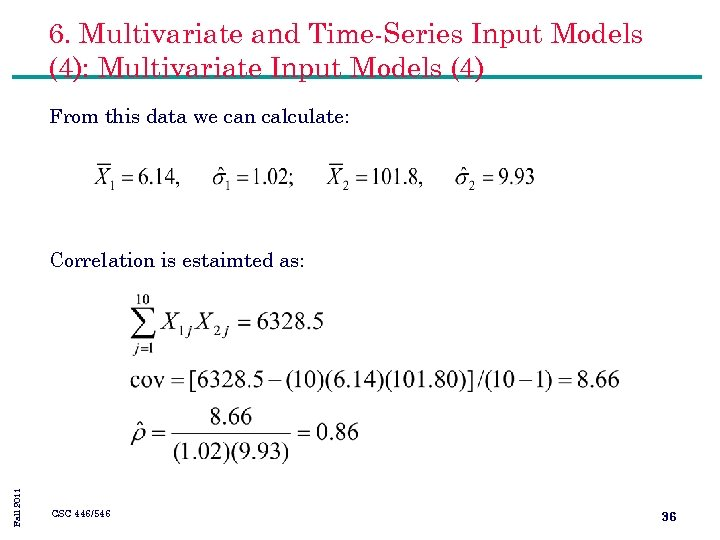6. Multivariate and Time-Series Input Models (4): Multivariate Input Models (4) From this data we can calculate: Fall 2011 Correlation is estaimted as: CSC 446/546 36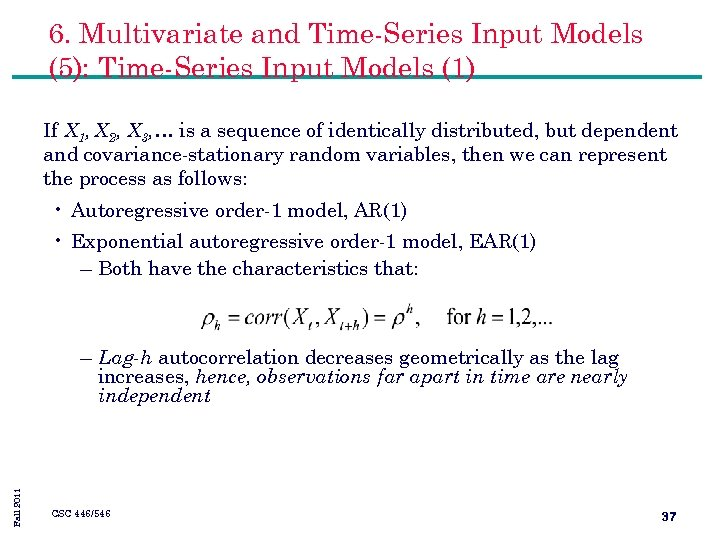6. Multivariate and Time-Series Input Models (5): Time-Series Input Models (1) If X 1, X 2, X 3, … is a sequence of identically distributed, but dependent and covariance-stationary random variables, then we can represent the process as follows: • Autoregressive order-1 model, AR(1) • Exponential autoregressive order-1 model, EAR(1) – Both have the characteristics that: Fall 2011 – Lag-h autocorrelation decreases geometrically as the lag increases, hence, observations far apart in time are nearly independent CSC 446/546 37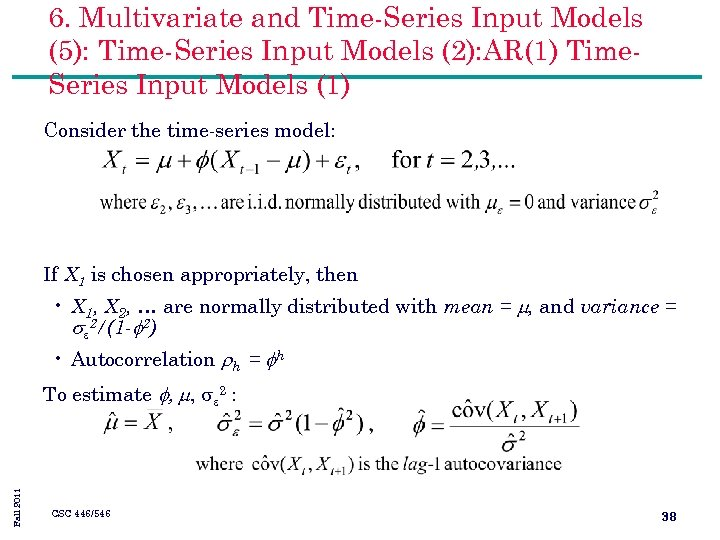6. Multivariate and Time-Series Input Models (5): Time-Series Input Models (2): AR(1) Time. Series Input Models (1) Consider the time-series model: If X 1 is chosen appropriately, then • X 1, X 2, … are normally distributed with mean = , and variance = se 2/(1 - 2) • Autocorrelation h = h Fall 2011 To estimate , , e 2 : CSC 446/546 38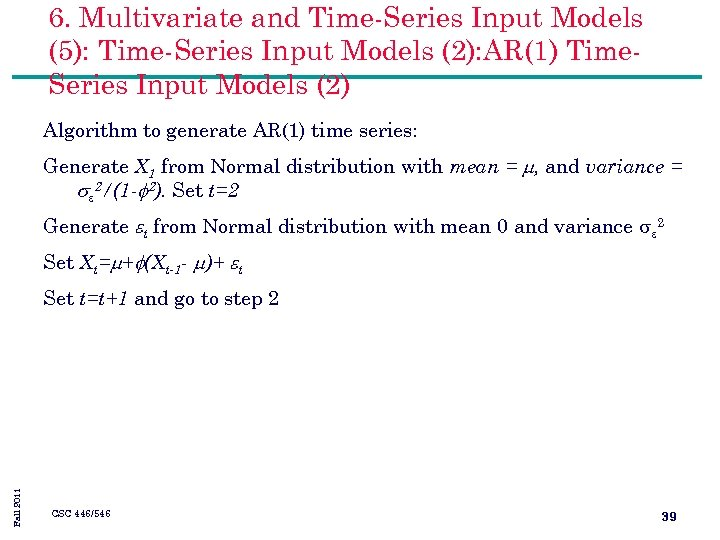6. Multivariate and Time-Series Input Models (5): Time-Series Input Models (2): AR(1) Time. Series Input Models (2) Algorithm to generate AR(1) time series: Generate X 1 from Normal distribution with mean = , and variance = se 2/(1 - 2). Set t=2 Generate t from Normal distribution with mean 0 and variance e 2 Set Xt= + (Xt-1 - )+ t Fall 2011 Set t=t+1 and go to step 2 CSC 446/546 39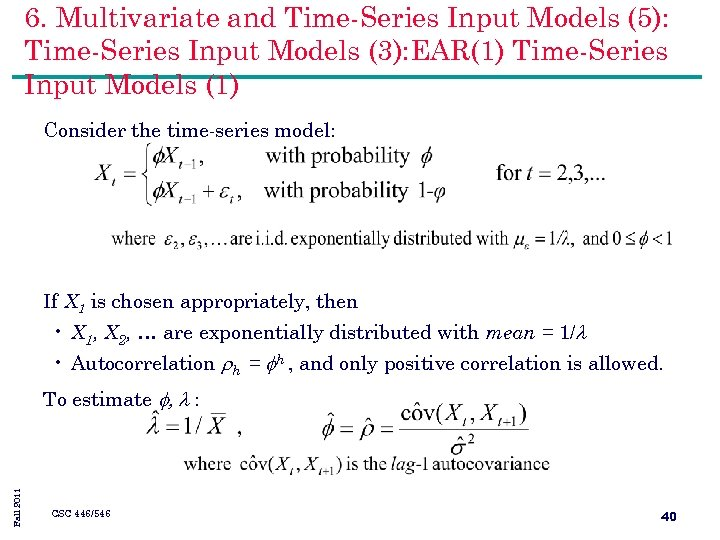6. Multivariate and Time-Series Input Models (5): Time-Series Input Models (3): EAR(1) Time-Series Input Models (1) Consider the time-series model: If X 1 is chosen appropriately, then • X 1, X 2, … are exponentially distributed with mean = 1/ • Autocorrelation h = h , and only positive correlation is allowed. Fall 2011 To estimate , : CSC 446/546 40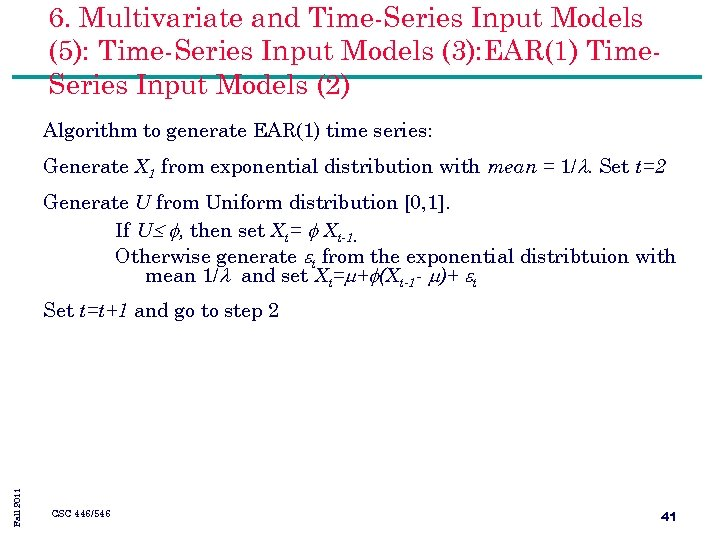6. Multivariate and Time-Series Input Models (5): Time-Series Input Models (3): EAR(1) Time. Series Input Models (2) Algorithm to generate EAR(1) time series: Generate X 1 from exponential distribution with mean = 1/. Set t=2 Generate U from Uniform distribution [0, 1]. If U , then set Xt= Xt-1. Otherwise generate t from the exponential distribtuion with mean 1/ and set Xt= + (Xt-1 - )+ t Fall 2011 Set t=t+1 and go to step 2 CSC 446/546 41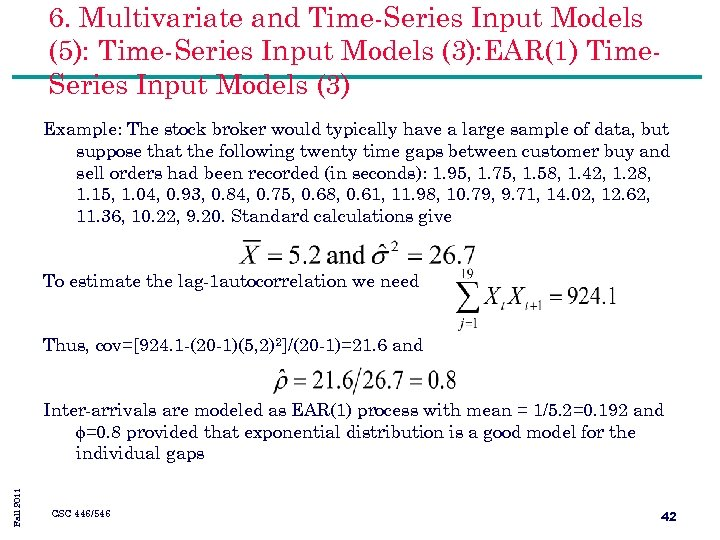6. Multivariate and Time-Series Input Models (5): Time-Series Input Models (3): EAR(1) Time. Series Input Models (3) Example: The stock broker would typically have a large sample of data, but suppose that the following twenty time gaps between customer buy and sell orders had been recorded (in seconds): 1. 95, 1. 75, 1. 58, 1. 42, 1. 28, 1. 15, 1. 04, 0. 93, 0. 84, 0. 75, 0. 68, 0. 61, 11. 98, 10. 79, 9. 71, 14. 02, 12. 62, 11. 36, 10. 22, 9. 20. Standard calculations give To estimate the lag-1 autocorrelation we need Thus, cov=[924. 1 -(20 -1)(5, 2)2]/(20 -1)=21. 6 and Fall 2011 Inter-arrivals are modeled as EAR(1) process with mean = 1/5. 2=0. 192 and =0. 8 provided that exponential distribution is a good model for the individual gaps CSC 446/546 42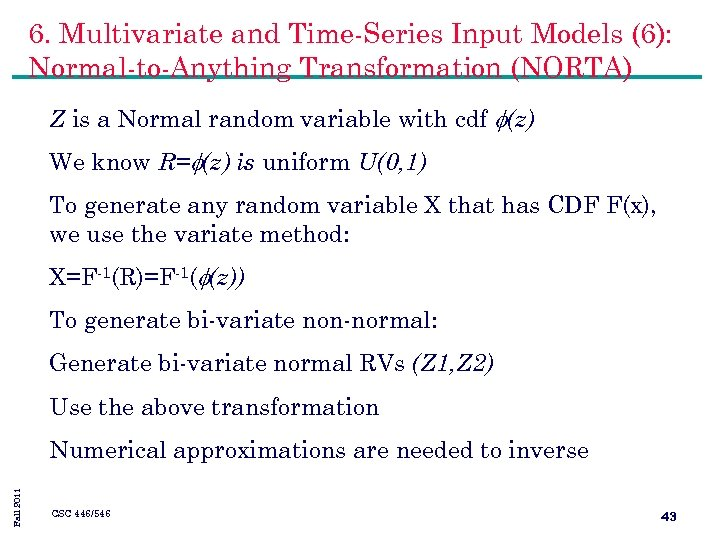6. Multivariate and Time-Series Input Models (6): Normal-to-Anything Transformation (NORTA) Z is a Normal random variable with cdf (z) We know R= (z) is uniform U(0, 1) To generate any random variable X that has CDF F(x), we use the variate method: X=F-1(R)=F-1( (z)) To generate bi-variate non-normal: Generate bi-variate normal RVs (Z 1, Z 2) Use the above transformation Fall 2011 Numerical approximations are needed to inverse CSC 446/546 43